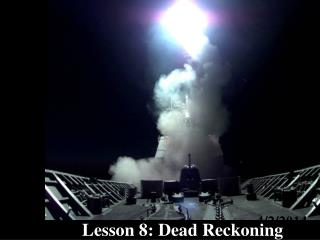Download Presentation# Lesson 8: Dead Reckoning - PowerPoint PPT PresentationDownload Presentation- - - - - - - - - - - - - - - - - - - - - - - - - - - E N D - - - - - - - - - - - - - - - - - - - - - - - - - - -
##### Presentation Transcript

1. Lesson 8: Dead Reckoning 4/2/2014

2. Lesson 7: Dead Reckoning • AGENDA: • Definition of Dead Reckoning • Six Rules of Dead Reckoning • Define/Apply the 3 min & 6 min Rules • Applicable reading: Hobbs pg. 134-138.

3. Dead Reckoning DR is the process of determining a ship’s approximateposition by applying, from a known position, a vector or a series of consecutive vectors representing the true courses steered and the distance run as determined by the ship’s speed and time,without considering the effects of wind and current.

4. Dead Reckoning • DR is derived from DEDUCED, or DED, reckoning which was the process by which a vessel’s position was computed trigonometrically in relation to a known point of departure. • This is performed as often as required by the 6 rules of DR • Uses: • Determine present position • Project future positions relative to hazards • Measure the effects of current (winds, seas) • Alter course to account the effects of current

5. Six Rules of DR

6. 0846 Plot a new Course Line with Every Fix or Running Fix c-075 s-15 0937 R-Fix c-106 s-10

7. Plot a DR Position Every Hour on the Hour 0900 1000 1100

8. Plot a DR Position atEvery Course Change 29 20 c-090 C-080 C-125 24

9. Plot a DR Position atEvery Speed Change 21 27 15 S-10 S-15 S-5

10. Plot a DR Position for Every Line of Position (LOP) c-195 s-10 Tank 0826 0826

11. 0846 Plot a DR Position for Every Fix or Running Fix 46 37 0937 R-Fix

12. Speed-Time-Distance Calculations • STD calculations can be made using a nautical slide rule, electronic calculator, set of pre-computed tables, or speed nomogram. D = S x T where: D = distance traveled S = speed in knots T = time in hours

13. Three Minute Rule • In 3 minutes a vessel travels in yards a distance 100 times its speed in knots. D = S x 100 where: D = distance traveled (yds) S = speed in knots

14. Six Minute Rule • In 6 minutes a vessel travels in miles a distance equal to its speed in knots divided by 10. D = S / 10 where: D = distance traveled (nm) S = speed in knots

15. HOMEWORK

16. REVIEW • What is Dead Reckoning? • What are the 6 Rules of DR?